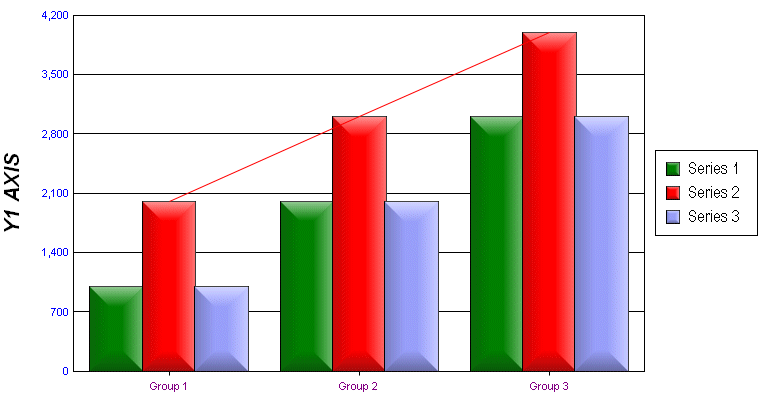# @MOVA (Moving Average Line)

This macro applies a scientific or standard moving average line to a specified series. Note that the bSciMovMode and nPeriod parameters are universal so they will apply to ALL moving average lines being drawn on the chart.

SYNTAX:

 `@MOVA nSeries bShow bSciMovMode nPeriod`

PARAMETERS:

nSeries; 0...999 series number (0=Series 1)

bShow; 1 = Show moving average. 0 = Hide moving average.

bSciMovMode; 1 = Scientfic moving average. 0 = Standard moving average

nPeriod; # of periods to use in moving average calculation.

EXAMPLE:

 ```@MOVA 1 1 1 1 ```PERSISTENT:

YES

ALSO SEE:

@TRENDLINE; to draw a trend line

@TRENDLINE_ALLDATA to draw a trend line through all data points

 ``` ```

Next MacroPrevious MacroReturn to Alpha List

Next Macro in this GroupPrevious Macro in this GroupReturn to Group List